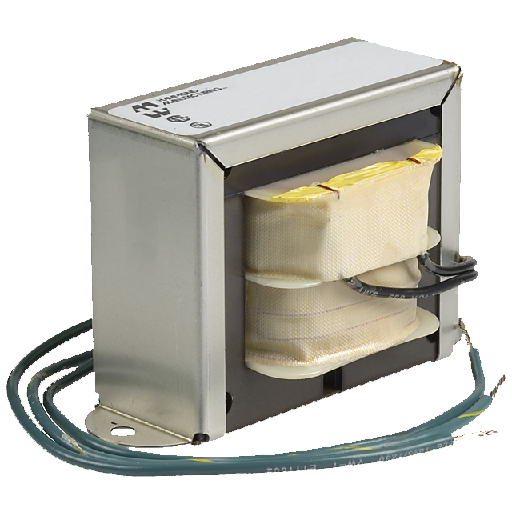# Transformer DesignerThis app is for transformer designing calculations. Transformers are widely used in our electrical applications to step down or step up AC voltage. These are used in power supplies, stabilizers, inverters, converters, UPS and so many other applications. This app is complete solution especially for 2 winding transformer. This app helps transformer designers to find out different transformer parameters. These parameters are

1. Turn/Volt
2. Turns for each coil
3. SWG/AWG Wire for each coil with actual rate (cmils/A)
4. Core Area for bobbin selection

During transformer design we need to know core area of transformer for the selection of bobbin. We want to calculate turns/volt so that we can calculate total turns for each coil. For each different coil different SWG/AWG wire is needed. We have to decide how much thick wire will be able to carry required amount of charge through it. Wires are divided according to their thickness/area of cross section. Each wire is assigned a number according to Standard Wire Gauge (SWG) or American Wire Gauge (AWG). This number is called SWG or AWG number of that wire. We know, a thick wire will be able to carry more charge than a thin wire. Here, I have considered circular mils (cmils) as unit of cross sectional area measurement.

This app is helpful in transformer winding, power supplies, inverters, converter and UPS development and sales business. Now, if you are a transformer designer/winder this app is for you. You can install it from here.### How It Works?

Let us consider we want a transformer for our LED sign board supply. LED sign board needs almost 12V and 4A supply. Considering other factors like temperature, we decide to design a transformer for 12V and 5A. Now, run this app and first enter 1 number of output coils like shown in the following figure and press “Next”.

Now, enter VA, Voltage and/or Current values as shown in the following figure. Here you have to enter first and the only output coil’s power requirement. Enter any two of the values and leave single value empty. If you entered all three values then app will calculate third according to left to right order means it will calculate “Current” value by dividing “VA” by “Voltage”. After this press “Next”.

Now, enter number of input coils. Here, in our example this is 1.

Here, enter “Voltage” or “Current” value of input coil but not both as total VA of transformer are calculated according to output coils and is shown in the following figure.

Now, enter value of Rate. In open air wires remain cool and can pass charge at more rate means with less cross sectional area a wire can pass more charge and so more current. But, when we use same wire in transformer/coil winding where wires are wound in layers, one layer over other and there is no air present between these layers but there is paper between them, so wires fail to get cool due to air, their charge passing capability is reduced and so, can pass charge at lower rates. Usually, we double rate (area) in transformers than in open air. We use 500/530 cmils/Amp value or more for transformers. There are so many other factors that affect this value, some of which are temperature, peak load time, working duration of machine per day etc. So, you have to choose better value of Rate considering all these factors. Here, we enter 530 cmils/A. Enter flux density of core used in weber per square inch. Default value for silicon core is 0.0006 weber per square inch. This app is optimized for silicon core. You can use other cores and do experiment. Finally, enter frequency of input voltage. In my country it is 50 Hz. Press “Next” to continue

Now, here results are calculated.

Here for each output and input coil following parameters are calculated

1. VA and/or Voltage and/or Current
2. Turns for coil
3. 2 SWG numbers with actual rate (in cmils/A)
4. 2 AWG numbers with actual rate (in cmils/A)

First SWG is safe SWG and second is calculated for your ease if it is with acceptable rate for you. Because this value also depend some other factors like how long transformer will be operated without resting and surrounding temperature etc. Usually we choose between 500 cmils/A and 530 cmils/A. Similarly, 2 AWG numbers are calculated. First is safe and second may or may not be safe for you. Now, scroll and see other parameters

At the end there are some other parameters of transformer like

1. Total VA of transformer
2. Turn/Volt
3. Core Area
4. Selected Rate value
5. Frequency and
6. Flux Density of core

### Disclaimer

We have designed this app at our best. We have tested it so many times but use it at your own risk. We will not responsible for any kind of loss in any form due to this app. If you find any bug or defect then please inform us at udigital.solutions@gmail.com. If you have a mind blowing idea of an app you can also share with us at this email.